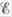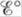Chapter 18, Problem 10ALQ

Chapter
Section
Textbook Problem

# What is the difference betweenand? When isequal to zero? When isequal to zero? (Consider “regular” galvanic cells as well as concentration cells.)

Interpretation Introduction

Interpretation:

The difference between E and E° and the condition at which galvanic cell and concentration cell have values of E and E° equal to zero is to be stated.

Concept introduction:

When there is a difference of potential between the electrodes, the flow of current occurs in the cell.

When there is potential difference between electrodes at standard conditions, it is called E° while at non-standard conditions, it is called E of the cell.

To determine: The difference between E and E° ; the condition at which E becomes zero and the condition at which E° become zero both for galvanic cell and concentration cell.

Explanation

The potential of the cell at the non-standard conditions is E and the value of cell potential at the standard conditions at the given temperature is E° .

The value of E° is determined with standard hydrogen electrode having cell potential equal to zero while E is determined using the Nernst equation.

The Nernst equation is defined as follows,

E = E° - (RTnF) ln(Q)

Where the following terms are defined as,

• E is the cell potential.
• E° is the cell potential at the standard conditions.
• R is the universal gas constant.
• T is the given temperature.
• F is the Faraday’s constant.
• Q is the activity of the species in the cell.
• n is the number of electrons involved in the reaction.

The value of E becomes equal to zero at equilibrium

### Still sussing out bartleby?

Check out a sample textbook solution.

See a sample solution

#### The Solution to Your Study Problems

Bartleby provides explanations to thousands of textbook problems written by our experts, many with advanced degrees!

Get Started